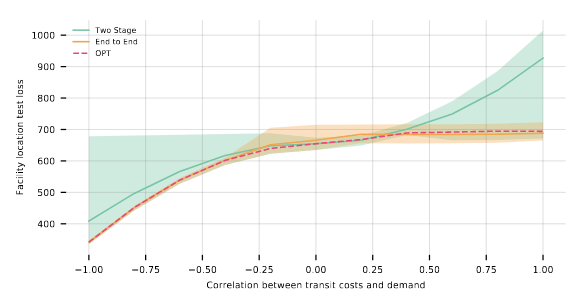Optimization Based Modelling

Optimization layers allow incorporating downstream optimization problems into neural network training. The performance gap between the first-learn-then-optimise and the end-to-end approaches in the perfect model setting is shown to be unbounded for non-linear cost functions.

This pill is inspired by the tutorial Differentiable Optimization: Integrating Structural Information Into Training Pipeline given at IJCAI 2022.

In decision-focused machine learning one wishes to compute a decision $z$ given an observation $x$ that minimizes the expected cost of a known function $f(y, z),$ where $y$ is drawn from an unknown distribution $P(Y | X=x).$ In other words, one aims to compute from $x$ the $z$ that minimizes $E_{y\sim P(Y|X=x)}[f(y, z)].$

Inference tasks with an optimization component are not uncommon. For example, a taxi company may want to position its cabs such that the total demand weighted by the travel times of the closest cabs to strategically important places in the city is minimized. The travel time and the demand might depend on some observable factors such as weather conditions or industrial fairs currently taking place.

Leveraging the known loss function of the downstream task can help to reduce the amount of training data. Classically, this has often been done with a two-step approach: A predictor $g(x)$ is learned to predict a surrogate $y$ given $x$ - usually $y = E[Y|X=x]$ - and the optimization then minimizes the deterministic objective $f(g(x), z)$. When $f$ is linear, it is easy to see that using the true conditional expectation of $y$ yields the optimal solution. However, for general $f$, using the cost with respect to the conditional expectation rather than the conditional expected costs (the expectation wrt. $P(Y|X)$) yields suboptimal results.

In recent years a new end-to-end alternative has emerged. With differentiable optimization layers it becomes possible to train the predictor $g$ directly on the downstream tasks by differentiating through the optimization tasks. This has been used for instance to incorporate safety constraints in learned controllers, see our pill on BarrierNet: A Safety-Guaranteed Layer for Neural Networks. Currently, the most mature implementation is probably cvxpylayers [Agr19D], which allows integrating convex optimization layers into models written in PyTorch, Jax, or Tensorflow (see here for an intuitive introduction).

[Cam22P], presented at AAAI 2022, investigates the potential performance gap between the two-step and the end-to-end approaches. Obviously, if the same predictor model is used then the optimal model that can be obtained by the end-to-end approach incurs a loss that is at most as high as the loss incurred by the two-step approach. By drawing connections to the price of correlation from stochastic optimization [Agr12P], they show that, in the perfect model setting, the ratio between the loss of the two-step approach and loss of the end-to-end approach can be unbounded for the true objective. This holds also in the practically relevant case where the non-linearity is in the multiplication of two components of y (as we have in our demand weighted traveling time).

Further they show that the end-to-end approach is optimal for two-stage minimal cost-flow problems, two-stage stochastic set cover, and monotone submodular cost functions. However, they also show that the end-to-end approach is not optimal in general.

The analysis is accompanied by a simulation study on a synthetic facility location problem that is very similar to our taxi example. By varying the correlation between the demand and travel time per location in the conditional distribution they demonstrate that the non-independence of the components of $y$ is the cause for the sub-optimality of the two-step approach on this type of cost functions.I think the possibility of incorporating downstream optimization tasks into the training of neural networks opens interesting opportunities. A possible next step could be to learn approximate conditional generative models through the optimization objective (possibly in combination with Monte-Carlo sampling). This way the model might be able to concentrate on learning the relevant covariance structure for the downstream task as precisely as possible. I would be interested in seeing how this compares to the corresponding two-step approach in practice.